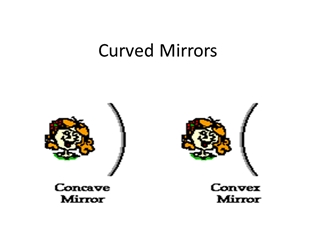# Curved Mirrors - Central Bucks School District

0

#### Presentation Transcript

• 1.Curved Mirrors
• 2.Do rays follow the law of reflection… For plane mirrors? For Concave Mirrors? For convex mirrors? For irregular reflective surfaces?
• 3.Do rays follow the law of reflection… For plane mirrors? YES! For Concave Mirrors? YES! For convex mirrors? YES! For irregular reflective surfaces? YES! First three cause specular reflection, the last causes diffuse reflection
• 4.Concave Mirrors and Rays
• 5.What affects the… distance to the focal point? distance to the image? size of the image? orientation of the image?
• 6.What affects the… distance to the focal point? (focal length) This is only affected by the radius of curvature of the mirror distance to the image? This is affected by the focal length and object distance size of the image? This is affected by the focal length, object distance and object size orientation of the image? This is determined based on focal lenth and object distance
• 7.Anatomy of a Concave Curved Mirror positive distances negative distances
• 8.What is the mathematical relationship between… …the image distance (cm) and the object distance (cm) for a spherical concave mirror?
• 9.What is the mathematical relationship between ________distance (m) and ___________distance (m) for a concave, spherical mirror?
• 10.The Mirror Equations 1/f = 1/do + 1/di M = hi/ho = -di/do f = focal length, it is POSITIVE for concave mirrors, and half of the radius of curvature M = magnification do = object distance di = image distance image distance is NEGATIVE when do < f and POSITIVE when do > f The image is real, inverted, and on the positive side of the mirror when do > f The image is virtual, upright, & on NEGATIVE side of the mirror when do < f
• 11.Example Determine the image distance and image height for a 10.0cm tall object placed 50.0 cm from a concave mirror having a focal length of 30.0 cm.
• 12.Rules for Rays:(for an object that is sitting on the principle axis) Draw a ray from the tip of the object toward the mirror that is parallel to the principle axis. This ray will be reflected thru the focus. Draw a ray from the tip of the object (along a line that passes) through the focus to the mirror. This ray is reflected parallel to the principle axis. The tip of the image is at the location that the reflected rays cross. The base of the image is on the principle axis. The image is perpendicular to the principle axis.
• 13.Ray Diagram for Concave Mirrors
• 14.More Ray Diagrams for Concave Mirrors
• 15.Still More Ray Diagrams!
• 16.And ONE MORE!
• 17.Helpful Assignment: PCR Lesson 3f CYU #1,2,5 (You should do more if you struggle with these three!) Skim Lessons 3a-3d
• 18.Fire from the Sun What was the object in the demo? What is the object distance? Due to the size of the object distance, 1/do is ALMOST…. Therefore, the image distance is pretty close to being……. In other words, If we had taken a meter stick outside (and used it), we would now know the….
• 19.Convex Mirrors
• 20.Ray Diagrams and Convex mirrors Principal Axis
• 21.The “Mirror Equation” 1/f = 1/do + 1/di f = focal length, it is NEGATIVE, and half of the radius of curvature do = object distance di = image distance, it is NEGATIVE For a CONVEX MIRROR, the image is always virtual, upright, and on the NEGATIVE side of the mirror.
• 22.Convex Mirror Example A convex mirror has a focal length of -50 cm. A 20 cm tall object is placed on the principal axis, 100 cm from the mirror's surface. Construct a ray diagram for this set-up. Determine the image distance from the diagram, then confirm your results with the mirror equation.
• 23.Culminating Experiment: Experimentally Determine the focal length of a concave mirror. State the objective Draw the set-up, labelling object, image, and distances (there are THREE) Present measurements and computed values in a table Show formulas, and “sample” calculations (with measurements plugged in) for one of the trials. Average result and state in a concluding sentence.